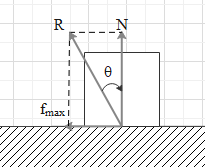QuestionAnswers

# The coefficient of friction between two surfaces is 0.2. The angle of friction is$\text{A}\text{. }{{\sin }^{-1}}(0.2)$\text{B}\text{. co}{{\text{s}}^{-1}}(0.2)$\text{C}\text{. ta}{{\text{n}}^{-1}}(0.1)$$\text{D}\text{. co}{{\text{t}}^{-1}}(5)$Hint: The angle of friction ($\theta$) is defined as the angle made by the resultant of the normal reaction and the maximum frictional force with the normal reaction. In this case, $\tan \theta =\dfrac{{{f}_{\max }}}{N}$ and ${{f}_{\max }}=\mu N$.

Formula used:
${{f}_{\max }}=\mu N$

Consider a block of mass m resting on a horizontal surface. Let the coefficient of friction between the surface of the block and the horizontal surface be $\mu$.
Friction is a force that opposes the relative motion between two surfaces. Therefore, if we try to move this block with a force, the frictional force between the block and horizontal surface will oppose the applied force. The direction of frictional force is always parallel to the surfaces in contact and opposite to the relative motion or force applied.
Force of friction is a variable force. The maximum value of the frictional force on the block is given by ${{f}_{\max }}=\mu N$, where N is the normal reaction on the block by the horizontal surface. In this case, the normal reaction on the block is in upward direction.
The angle between the resultant of the maximum force of friction and the normal reaction and the normal reaction is called angle of friction.Let us find the value of the angle of friction. Let the angle be $\theta$.
If you see the given figure, $\tan \theta =\dfrac{{{f}_{\max }}}{N}$ …… (i).
And we know that ${{f}_{\max }}=\mu N$. Substitute this value in equation (i).
$\Rightarrow \tan \theta =\dfrac{\mu N}{N}$.
$\Rightarrow \tan \theta =\mu$
This means the tangent of the angle of friction between two surfaces is equal to the coefficient of friction between the two surfaces.
In the given question, $\mu$=0.2.
Hence, $\Rightarrow \tan \theta =0.2$.
The reciprocal of $\tan \theta$ is $\cot \theta$.
$\Rightarrow \cot \theta =\dfrac{1}{\tan \theta }=\dfrac{1}{0.2}$.
$\Rightarrow \cot \theta =\dfrac{1}{\tan \theta }=\dfrac{1}{0.2}=5$
$\Rightarrow \theta ={{\cot }^{-1}}(5)$
This means the angle of friction between the given two surfaces is ${{\cot }^{-1}}(5)$.
Hence, the correct answer is option D.

Note:
Students may confuse between angle of friction and angle of repose.
We already know what angle of friction is.
Angle of repose is the minimum angle of inclination of a surface on which a block begins to slide under the gravitational force.
View Notes
Relationship Between Force of Limiting Friction and Normal ReactionStatic FrictionRolling Friction and Rolling ResistanceCBSE Class 11 Physics Law of Motion FormulasCBSE Class 11 Physics Thermal Properties of Matter FormulasCBSE Class 11 Physics Mechanical Properties of Solids FormulasCoefficient of DeterminationCBSE Class 11 Physics Mechanical Properties of Fluids FormulasCBSE Class 11 Physics Systems of Particles and Rotational Motion FormulasKarl Pearson’s Coefficient of CorrelationCBSE Class 12 Physics Question Paper 2020Previous Year Question Paper for CBSE Class 12 PhysicsPrevious Year Question Paper for CBSE Class 12 Physics - 2015Previous Year Question Paper for CBSE Class 12 Physics - 2018Previous Year Question Paper for CBSE Class 12 Physics - 2014Previous Year Question Paper for CBSE Class 12 Physics - 2013Previous Year Question Paper for CBSE Class 12 Physics - 2019Physics Question Paper for CBSE Class 12 - 2016 Set 1 CPrevious Year Physics Question Paper for CBSE Class 12 - 2017Previous Year Question Paper of CBSE Class 10 EnglishHC Verma Class 11 Physics Part-1 Solutions for Chapter 6 - FrictionFriction NCERT Solutions - Class 8 ScienceFriction NCERT Solutions - Class 8 ScienceNCERT Exemplar for Class 8 Science Solutions Chapter 12 FrictionNCERT Exemplar for Class 11 Physics Chapter 11 - Thermal Properties of Matter (Book Solutions)NCERT Solutions for Class 11 Physics Chapter 11 Thermal Properties of Matter in HindiClass 11 Physics NCERT solutionsNCERT Solutions for Class 11 Physics Chapter 5 Law of Motion in HindiNCERT Solutions for Class 8 Science Chapter 12 Friction in HindiNCERT Exemplar for Class 11 Physics Chapter 10 - Mechanical Properties of Fluids (Book Solutions)